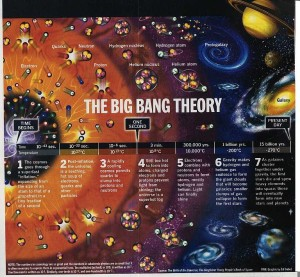Science Seen Physicist and Time One author Colin Gillespie helps you understand your world.

# The universe has an arithmetic of its own that answers a deep question about the nature of math.

Here’s a burning question behind the facade of math: Is math a property of the universe that we discover (a view philosophy calls realism); or is math an invention of our minds (fictionalism)?

If “our” math is a property of the universe then we may get it right or wrong but we can’t change it; if it is an invention of our minds then, if we don’t like it we can invent something different.

Math is the foundation of physics, the fundamental science and driver of the economy. Uncertainty about the very nature of mathematics weakens physics, infects all the sciences, and affects our lives and livelihoods.

For example there is deep disagreement about the nature of simple numbers. Great mathematicians set out to build a theory of simple arithmetic with elaborate logical devices. They all failed. In consequence, physics is riddled with inconsistency.Into this esoteric issue now steps the infant universe: Recent discoveries in particle physics combined with reinvigorated insights from the earliest days of cosmology show how to solve dozens of intractable problems with a simple cosmic beginning (far simpler than the messy, inconsistent Big Bang theory).

The laws of physics and arithmetic began with the beginning of the universe.

That simple beginning had no matter—not even a single subatomic particle—and no energy. It had just a single tiny Planck-sized fleck or quantum of space.

That fleck had (we must assume) three simple properties. It had six internal dimensions (as many string theories now assume). It had a volume (the Planck volume, which is why space is 3D). And, as space was highly curved, it was unstable; in a Planck-time instant it replicated itself and became two flecks.

Already we can see the universe enacting the basic objects of arithmetic. With the first fleck, we have the integer 1. And with the arrival of another, we have both addition + and, since they are identical, we have equals =. As two follows one, we also have the successor, or next integer, denoted by S.

Next instant the two flecks replicated to become four, so we have multiplication ×. (How this replication continued for a fraction of a second and twisted links gave rise to vast amounts of matter is another story.)

is a property of the universe: these four basic objects (1, +, S, ×) and all the integers that can be made with them up to the number of flecks the universe has so far made, a number with roughly 400 decimal digits.

For his famous Incompleteness Theorems (perhaps the most famous theorems ever) Kurt Gödel required arithmetic to have these same four mathematical objects plus one other, the zero 0. The distressing conclusion from these theorems was that the search to set arithmetic (and so physics) on a firm logical foundation was doomed to always fail; any such arithmetic must always be either inconsistent or incomplete.

But wait—the universe contains no zero! Can Gödel’s theorems be proved without a zero? No-one has done this and my bet is: It can’t.

Nor does the universe contain the real numbers. The space and time continua that set the stage for modern physics do not exist (as Bernhard Riemann suspected in the mid-1800s and is now widely recognized).

Nor does the universe, being finite after growing for a finite time, contain any infinity.

The travails with zeros and infinities that bedevil modern physics stand revealed for what they are: symptoms of assumptions that do not reflect reality.

So the universe’s answer to math’s burning question is: both. There is math that is a property of the universe. And there is math that we invent.

This does not make one good and the other bad. Both are useful. But they may be much more useful if we take care to distinguish between math fact and math fiction.

Today’s physics is based on the latter. A modest investment in studying math fact might yield a big return by way of new and better physics.

Image credits:

http://images.cryhavok.org/v/Big+Bang+Timeline.jpg.html

https://s-media-cache-ak0.pinimg.com/originals/16/09/f3/1609f3dd7e0a389e6aa7f095bda471cc.jpg